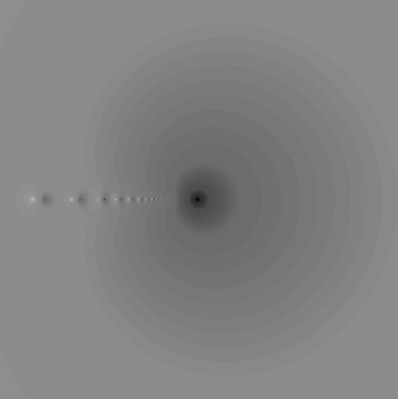www.johngill.net

 Mathematicae Varietas

6

Continued Fractions - Analytic Theory

Continued Fractions are algebraic constructions of the formIf this process does not terminate, the CF is said to be infinite, in the same way a non-terminating series is said to be infinite.  If the a(n)s and b(n)s are functions of a complex variable, z , and ω is another such variable, we haveCalled the normal nth convergent of the infinite CF when ω = 0.  Simpler notational formats include the following:It is possible to expand complex functions as continued fractions using a number of distinct algorithms. This variety of expansion formats, plus a bewildering assortment of convergence theorems, contrasts strongly with the relative simplicity of power series expansions and their convergence criteria. Why then bother with CFs? Two reasons: (1) frequently CF expansions converge more rapidly than the series (although not always), and, (2) CF expansions may be valid in a much larger domain than power series. As a simple - even trivial - example,The series on the left converges in a disc about the origin of radius 1. It diverges outside this disc. The expression on the right is, in fact, a finite CF, converging instantly for all values of  z  with the exception of  z = 1.

We begin with graphics, looking at a convergent of the periodic CF,Going down to the nth level. This convergent is a rational function of degree n, having both fixed points and poles. Here we see how the nth convergent displaces points in the z-plane, with little or no displacement = dark and high displacement = light:The point z = 0 is, of course, a fixed point ( F(z) = z ). The graph of the 50th convergent shows a series of alternating poles and what appear to be fixed points along the negative real axis (a portion of which is actually the branch line for the single valued function of which this is a CF expansion).  The image is centered at  z = 0  and extends 2 units to the right and left.

Next, we expand the function  F(z) = p.v. √z , about z = 0, obtaining another periodic CF:Comparing graphically the 10th convergent with the value of the function F(z), we have the following, where darkest hues indicate strong approximations (<10^-5), and light hues, poor approximations:The graph is centered at the origin, extending 10 units to the right and left. The branch line of the function extends from the origin along the negative real axis. Poles of the rational approximation appear faintly as light spots along the branch line.